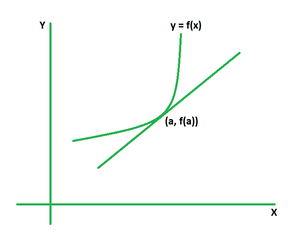Open in App
Not now

# Linear Approximation Formula

• Difficulty Level : Easy
• Last Updated : 26 Apr, 2022

A linear approximation is a mathematical term that refers to the use of a linear function to approximate a generic function. It is commonly used in the finite difference method to create first-order methods for solving or approximating equations. The linear approximation formula is used to get the closest estimate of a function for any given value.

Linear Approximation Formula

The linear approximation of a function is defined as using a line to approximate the function’s value at a given position. The concept of the tangent line is linked with the curve of a function with a point on it. The value of the function at any point that is very close to the provided point can be approximated using the equation of the tangent line if the equation of the tangent line is found at a given point. This notion is known as linear approximation, and it is also known as tangent line approximation because it is done using the tangent line.

Formula

Suppose a tangent line is drawn to the curve y = f(x) at the point (a, f(a)).The equation of tangent is the required linear approximation formula. It can be derived by using the point-slope form as its slope is the derivative of function f(x) at x = a, that is, f ‘(a).

L(x) = f(a) + f ‘(a) (x – a)

where,

L(x) is the linear approximation of the function f(x) for x = a,

f'(a) is the first derivative of f(x) for x = a.

### Sample Problems

Problem 1. Find the linear approximation of the function f(x) = x3 if the value of x is approaching 2.

Solution:

We have, f(x) = x3.

Now, f'(x) = d(f(x))/dx

= 3x2

For a = 2,

f(a) = 23 = 8

f'(a) = 3 (2)2 = 3 (4) = 12

Using the formula we have,

L(x) = f(a) + f ‘(a) (x – a)

= 8 + 12 (x – 2)

= 8 + 12x – 24

= 12x – 16

Problem 2. Find the linear approximation of the function f(x) = √x if the value of x is approaching 4.

Solution:

We have, f(x) = √x.

Now, f'(x) = d(f(x))/dx

= 1/(2√x)

For a = 4,

f(a) = √4 = 2

f'(a) = 1/(2√4) = 1/4

Using the formula we have,

L(x) = f(a) + f ‘(a) (x – a)

= 2 + (1/4) (x – 4)

= 2 + (x – 4)/4

= (x + 4)/4

Problem 3. Find the linear approximation of the function f(x) = sin x if the value of x is approaching π/3.

Solution:

We have, f(x) = sin x.

Now, f'(x) = d(f(x))/dx

= cos x

For a = π/3,

f(a) = sin π/3 = √3/2

f'(a) = cos π/3 = 1/2

Using the formula we have,

L(x) = f(a) + f ‘(a) (x – a)

= √3/2 + (1/2) (x – π/3)

= (3 (√3 + x) – π)/6

Problem 4. Find the linear approximation of the function f(x) = log x if the value of x is approaching 1.

Solution:

We have, f(x) = log x.

Now, f'(x) = d(f(x))/dx

= 1/x

For a = 1,

f(a) = log 1 = 0

f'(a) = 1/1 = 1

Using the formula we have,

L(x) = f(a) + f ‘(a) (x – a)

= 0 + 1 (x – 1)

= x – 1

Problem 5. Find the linear approximation of the function f(x) = tan x if the value of x is approaching π/3.

Solution:

We have, f(x) = tan x.

Now, f'(x) = d(f(x))/dx

= sec2 x

For a = π/3,

f(a) = tan π/3 = √3

f'(a) = sec2 π/3 = 4

Using the formula we have,

L(x) = f(a) + f ‘(a) (x – a)

= √3 + (4) (x – π/3)

=  (3 (√3 + 4x) – 4π)/3

My Personal Notes arrow_drop_up
Related Articles